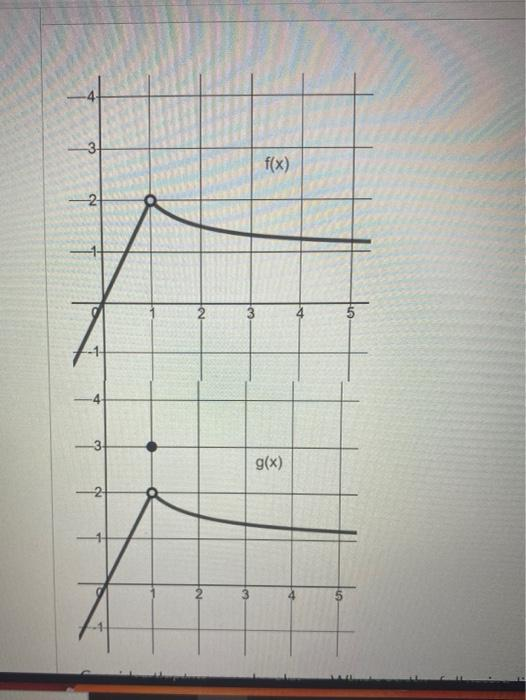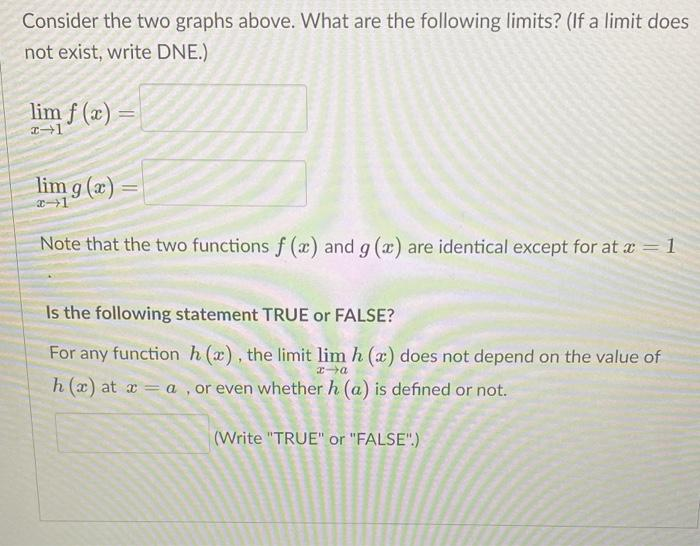# Question Solved1 Answer3 f(x) Na 2 -3 -3 g(x) -2 Consider the two graphs above. What are the following limits? (If a limit does not exist, write DNE.) lim f (x) 21 lim g(x) -1 Note that the two functions f (x) and g(x) are identical except for at x = 1 Is the following statement TRUE or FALSE? For any function h (2), the limit lim h (x) does not depend on the value of h(x) at x = a , or even whether h (a) is defined or not. (Write "TRUE" or "FALSE".).Transcribed Image Text: 3 f(x) Na 2 -3 -3 g(x) -2 Consider the two graphs above. What are the following limits? (If a limit does not exist, write DNE.) lim f (x) 21 lim g(x) -1 Note that the two functions f (x) and g(x) are identical except for at x = 1 Is the following statement TRUE or FALSE? For any function h (2), the limit lim h (x) does not depend on the value of h(x) at x = a , or even whether h (a) is defined or not. (Write "TRUE" or "FALSE".).
More
Transcribed Image Text: 3 f(x) Na 2 -3 -3 g(x) -2 Consider the two graphs above. What are the following limits? (If a limit does not exist, write DNE.) lim f (x) 21 lim g(x) -1 Note that the two functions f (x) and g(x) are identical except for at x = 1 Is the following statement TRUE or FALSE? For any function h (2), the limit lim h (x) does not depend on the value of h(x) at x = a , or even whether h (a) is defined or not. (Write "TRUE" or "FALSE".).